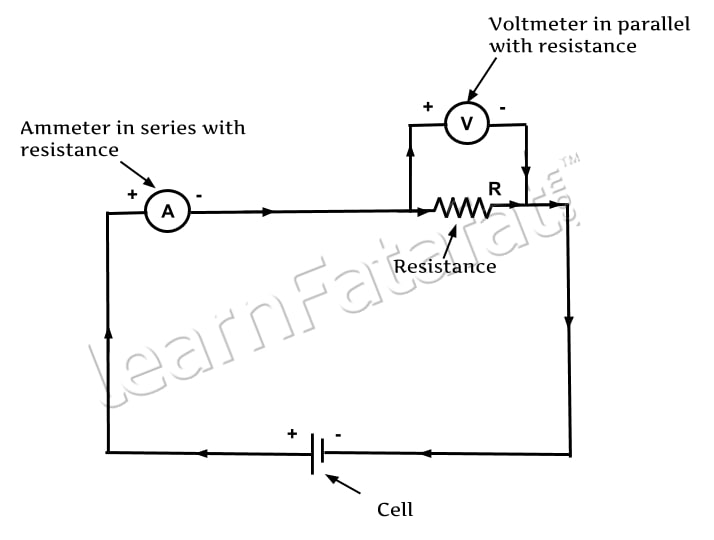# Class 10 Science Chapter 4 Electric Spark

### Electric Current

Electric current or Electricity is important in day to day life. Consider, movement of electrons in wire. When metallic wire is not connected to a battery, the free electrons move in random direction. Metallic conductor consist of large number of free electrons, these electrons are very loosely bonded to nucleus of atom and hence they move free. When metal wire is connected to battery, free electrons which are negatively charged moves to positive terminal of battery. This results into the electric current in the circuit. Electric current is defined as,

“Rate of flow of electric charges.”

Let Q be the total charge flowing through the wire in time t, therefore, electric current is given as I = Q/t. unit of electric current is Ampere and it is denoted by A. However, small currents are expressed in milli ampere mA and microampere μA.

### One Ampere current

The current is said to be one ampere if one coulomb of charge is flowing through a conductor in one second.
1A = 1C / 1s

### Electrical Circuit

Electrical circuit is consist of wires, battery, switch, bulb connected in a closed path through which the current flows. Circuits are represented by drawing electrical symbols on paper. Such diagram is called as circuit diagram.
Here are few symbols used in electric circuitElectric Circuit Symbols

A typical electric circuit consist of cell, an ammeter connected in series with the resistor, a voltmeter connected in parallel with resistor. The direction of current is from positive terminal to negative terminal.Electric Circuit

### Electric Potential

Electric potential is electrical level at a particular point. High electric potential is denoted by + sign and low potential is denoted by – sign. Current always flows from high potential to low potential.

### Potential difference

It is defined as, “Work done to move unit positive charge from one point to another in an electric field. ” the SI unit of potential difference is volts denoted by V. smaller values of potential difference are measured in mV and μV. However, larger values are measured in KV and MV. potential difference can be measured as P.D. = work done / Quantity of charge transferred.
P.D. = W/ Q

### One Volt Potential Difference

Potential difference is one volt when one joule of work is done in moving one coulomb of charge from one point to another in an electric field. 1V = 1J/ 1C

### Resistance

Electric current flows from negative terminal to positive terminal. While moving from negative terminal to positive terminal electrons collide with other electrons, atoms and ions in the conductor. These collisions oppose the flow of electron. The property of the conductor to oppose the current flowing through it is called as resistance. Resistance is equal to ratio of potential difference across the ends of conductor to the current flowing through the conductor. The SI unit of resistance is ohm and is denoted by Ω.

### One Ohm Resistance

The resistance is said to be one ohm when one ampere of current flows through a conductor having one volt of potential difference.
1Ω = 1V / 1A.### Conductors and Insulators

Substances having very low resistance is called as conductors. For example, Copper, Aluminium, Silver, Gold.
In contrast, substances having very high resistance are called as insulators. For example, Rubber, Wood, Glass.

Keywords: Ampere, Volts, Potential Difference, Conductors, Insulators, Ohm

Share Fatafat
• 1
•
•
•
•
1
Share